# Probability theory

Probability theory is the branch of mathematics concerned with probability. Although there are several different probability interpretations, probability theory treats the concept in a rigorous mathematical manner by expressing it through a set of axioms. Typically these axioms formalise probability in terms of a probability space, which assigns a measure taking values between 0 and 1, termed the probability measure, to a set of outcomes called the sample space. Any specified subset of the sample space is called an event.Central subjects in probability theory include discrete and continuous random variables, probability distributions, and stochastic processes (which provide mathematical abstractions of non-deterministic or uncertain processes or measured quantities that may either be single occurrences or evolve over time in a random fashion).Although it is not possible to perfectly predict random events, much can be said about their behavior. Two major results in probability theory describing such behaviour are the law of large numbers and the central limit theorem. As a mathematical foundation for statistics, probability theory is essential to many human activities that involve quantitative analysis of data. Methods of probability theory also apply to descriptions of complex systems given only partial knowledge of their state, as in statistical mechanics or sequential estimation. A great discovery of twentieth-century physics was the probabilistic nature of physical phenomena at atomic scales, described in quantum mechanics. (Wikipedia).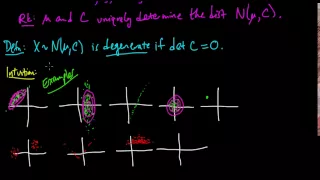(PP 6.2) Multivariate Gaussian - examples and independence

Degenerate multivariate Gaussians. Some sketches of examples and non-examples of Gaussians. The components of a Gaussian are independent if and only if they are uncorrelated.

From playlist Probability Theory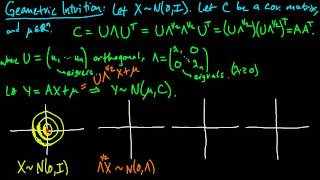(PP 6.6) Geometric intuition for the multivariate Gaussian (part 1)

How to visualize the effect of the eigenvalues (scaling), eigenvectors (rotation), and mean vector (shift) on the density of a multivariate Gaussian.

From playlist Probability Theory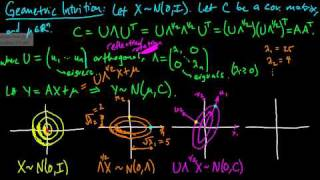(PP 6.7) Geometric intuition for the multivariate Gaussian (part 2)

How to visualize the effect of the eigenvalues (scaling), eigenvectors (rotation), and mean vector (shift) on the density of a multivariate Gaussian.

From playlist Probability Theory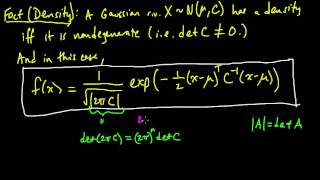(PP 6.4) Density for a multivariate Gaussian - definition and intuition

The density of a (multivariate) non-degenerate Gaussian. Suggestions for how to remember the formula. Mathematical intuition for how to think about the formula.

From playlist Probability Theory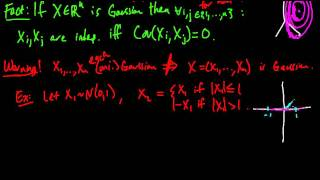(PP 6.3) Gaussian coordinates does not imply (multivariate) Gaussian

An example illustrating the fact that a vector of Gaussian random variables is not necessarily (multivariate) Gaussian.

From playlist Probability Theory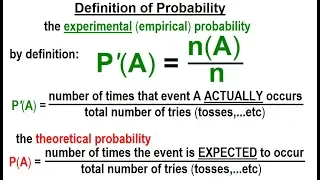Statistics: Ch 4 Probability in Statistics (20 of 74) Definition of Probability

Visit http://ilectureonline.com for more math and science lectures! To donate: http://www.ilectureonline.com/donate https://www.patreon.com/user?u=3236071 We will learn the “strict” definition of experimental (empirical) and theoretical probability. Next video in this series can be seen

From playlist STATISTICS CH 4 STATISTICS IN PROBABILITY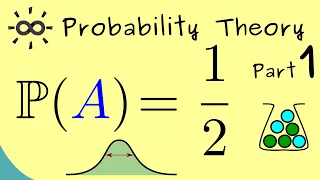Probability Theory - Part 1 - Introduction (including R)

Support the channel on Steady: https://steadyhq.com/en/brightsideofmaths Or support me via PayPal: https://paypal.me/brightmaths Or via Ko-fi: https://ko-fi.com/thebrightsideofmathematics Or via Patreon: https://www.patreon.com/bsom Or via other methods: https://thebrightsideofmathematics.

From playlist Probability Theory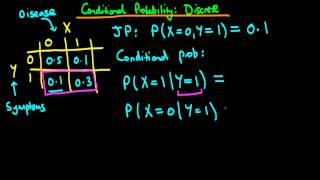What is a conditional probability?

An introduction to the concept of conditional probabilities via a simple 2 dimensional discrete example. If you are interested in seeing more of the material, arranged into a playlist, please visit: https://www.youtube.com/playlist?list=PLFDbGp5YzjqXQ4oE4w9GVWdiokWB9gEpm For more inform

From playlist Bayesian statistics: a comprehensive course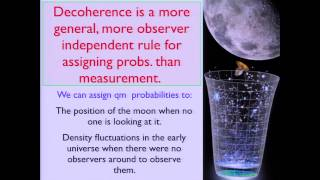The State of the Universe - J. Hartle - 12/9/2013

A conference celebrating the 50th anniversary of quarks honoring Murray Gell-Mann was held at Caltech on December 9-10, 2013. For more information, visit: http://hep.caltech.edu/gm/Probability & Information Theory — Subject 5 of Machine Learning Foundations

#MLFoundations #Probability #MachineLearning Welcome to my course on Probability and Information Theory, which is part of my broader "Machine Learning Foundations" curriculum. This video is an orientation to the curriculum. There are eight subjects covered comprehensively in the ML Found

From playlist Probability for Machine LearningMath Major Guide | Warning: Nonstandard advice.

A guide for how to navigate the math major and how to learn the main subjects. Recommendations for courses and books. Comment below to tell me what you think. And check out my channel for conversation videos with guests on math and other topics: https://www.youtube.com/channel/UCYLOc-m8Wu

From playlist Math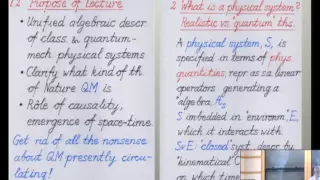Quantum Mechanics -- a Primer for Mathematicians

Juerg Frohlich ETH Zurich; Member, School of Mathematics, IAS December 3, 2012 A general algebraic formalism for the mathematical modeling of physical systems is sketched. This formalism is sufficiently general to encompass classical and quantum-mechanical models. It is then explained in w

From playlist Mathematics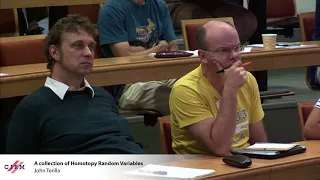John Terilla : A collection of Homotopy Random Variables

Recording during the thematic meeting : "Geometrical and Topological Structures of Information" the August 29, 2017 at the Centre International de Rencontres Mathématiques (Marseille, France) Filmmaker: Guillaume Hennenfent

From playlist Geometry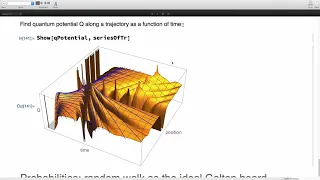Using Computational Essays to Demystify Quantum Mechanics

To learn more about Wolfram Technology Conference, please visit: https://www.wolfram.com/events/technology-conference/ Speaker: Mohammad Bahrami Wolfram developers and colleagues discussed the latest in innovative technologies for cloud computing, interactive deployment, mobile devices,

From playlist Wolfram Technology Conference 2018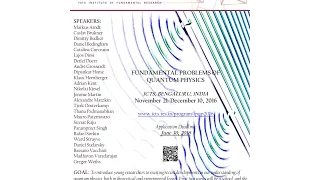The measurement problem and some mild solutions by Dustin Lazarovici (Lecture - 04)

21 November 2016 to 10 December 2016 VENUE Ramanujan Lecture Hall, ICTS Bangalore Quantum Theory has passed all experimental tests, with impressive accuracy. It applies to light and matter from the smallest scales so far explored, up to the mesoscopic scale. It is also a necessary ingredie

From playlist Fundamental Problems of Quantum Physics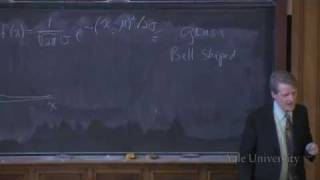2. The Universal Principle of Risk Management: Pooling and the Hedging of Risks

Financial Markets (ECON 252) Statistics and mathematics underlie the theories of finance. Probability Theory and various distribution types are important to understanding finance. Risk management, for instance, depends on tools such as variance, standard deviation, correlation, and regr

From playlist Financial Markets (2008) with Robert ShillerQuantum Theory - the Born Interpretation: Oxford Mathematics 2nd Year Student Lecture

Here is the latest lecture in the series of undergraduate lectures that we are making available to give an insight in to life in Oxford Mathematics. This lecture from James Sparks is taken from his Second Year Quantum Theory course. So far in the course we've met the Schrodinger equation

From playlist Oxford Mathematics 2nd Year Student Lectures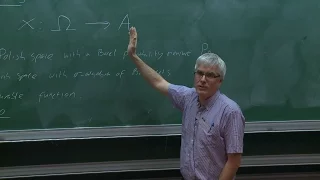Alex SIMPSON - Probability sheaves

In , Tao observes that the probability theory concerns itself with properties that are \preserved with respect to extension of the underlying sample space", in much the same way that modern geometry concerns itself with properties that are invariant with respect to underlying symmetries

From playlist Topos à l'IHES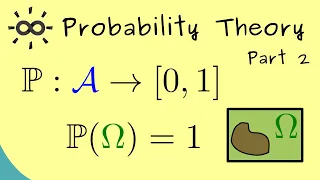Probability Theory - Part 2 - Probability Measures

Support the channel on Steady: https://steadyhq.com/en/brightsideofmaths Or support me via PayPal: https://paypal.me/brightmaths Or via Ko-fi: https://ko-fi.com/thebrightsideofmathematics Or via Patreon: https://www.patreon.com/bsom Or via other methods: https://thebrightsideofmathematics.

From playlist Probability Theory